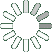Puzzle Command
Daily Puzzle
Number Logic #9
December 28, 2014Find a 6 digit number where:

The 1st digit is the number of 3s in the number.
The 2nd digit is higher than 2.
The 3rd digit is higher than 2.
The 4th digit is the sum of the 1st and 2nd digit.
The 5th digit is the sum of the 2nd and 1st digit.
The 6th digit is the 7.

Every digit is higher than 2.

Puzzle Notes
6 digit numbers range from 100,000 to 999,999.
Enter in your solution below and click submit.